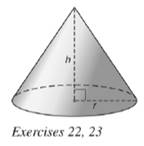Chapter 9.3, Problem 22E### Elementary Geometry for College St...

6th Edition
Daniel C. Alexander + 1 other
ISBN: 9781285195698

#### Solutions

Chapter
Section### Elementary Geometry for College St...

6th Edition
Daniel C. Alexander + 1 other
ISBN: 9781285195698
Textbook Problem
1 views

# For the accompanying right circular cone, h = 6 m and r = 4 m. Find the exact and approximatea) lateral area.b) total area.c) volume.To determine

(a)

To find: The exact and approximate lateral area of the right circular cone.

Explanation

Given:

Consider a right circular cone with h=6 m and r=4 m.

Formula used:

In a right circular cone r2+h2=l2.

The lateral area of the right circular cone is L=πrl

Calculation:

We have h=6 m and r=4 m.

Substitute the known values in the formula,

r2+h2=l242+62=l216+36=l2<

To determine

(b)

To find: The exact and approximate total area of the right circular cone.

To determine

(c)

To find: The exact and approximate volume of the right circular cone.

### Still sussing out bartleby?

Check out a sample textbook solution.

See a sample solution

#### The Solution to Your Study Problems

Bartleby provides explanations to thousands of textbook problems written by our experts, many with advanced degrees!

Get Started

#### Evaluate the integral, if it exists. x+2x2+4xdx

Single Variable Calculus: Early Transcendentals

#### Which graph represents y=x+1x

Study Guide for Stewart's Single Variable Calculus: Early Transcendentals, 8th

#### Identify the basic features of a between-subjects research design.

Research Methods for the Behavioral Sciences (MindTap Course List)